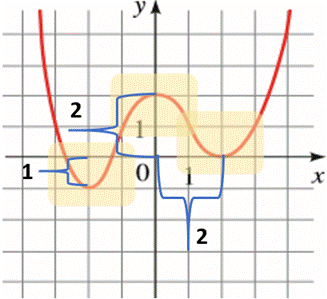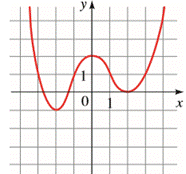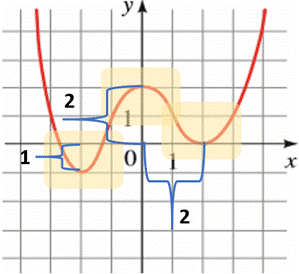# To find all the local maximum and minimum value of the function and the value of x at which each occur using the graph of the function given.### Precalculus: Mathematics for Calcu...

6th Edition
Stewart + 5 others
Publisher: Cengage Learning
ISBN: 9780840068071### Precalculus: Mathematics for Calcu...

6th Edition
Stewart + 5 others
Publisher: Cengage Learning
ISBN: 9780840068071

#### Solutions

Chapter 2.3, Problem 31E

a.

To determine

## To find all the local maximum and minimum value of the function and the value of x at which each occur using the graph of the function given.

Expert Solution

The local maximum and minimum value of the function are 2 and 1,0 respectively and the value of x at which each occur are 0 and 2,2 respectively.

### Explanation of Solution

Given information :

The graph of the function is given.Draw a viewing rectangle on each of the extremum of the graph of the function which is provided in the question.

The graph is shown below:From the above graph, it can be observed that the point (0,f(0)) is the highest point on the graph of f within the viewing rectangle, so the number f(0)=2 is a local maximum value of the function.

Similarly, it can be observed that the point (2,f(2)) and (2,f(2)) are the lowest points on the graph of f within the viewing rectangle, so the numbers f(2)=1 and f(2)=0 are the local minimum value of the function.

Hence,

The local maximum and minimum value of the function are 2 and 1,0 respectively and the value of x at which each occur are 0 and 2,2 respectively.

b.

To determine

### To find the interval on which the function is increasing and on which the function is decreasing.

Expert Solution

The function f is decreasing on (,2] and [0,2] and increasing on [2,0] and [2,) .

### Explanation of Solution

Given information :

The graph of the function is given.Concept used:

The function is increasing on an interval I if f(x1)<f(x2) whenever x1<x2 in I .

The function is decreasing on an interval I if f(x1)>f(x2) whenever x1<x2 in I .

The graph of the function is shown below:Use definition of increasing and decreasing function.

From the above graph, it can be observed that the function f is decreasing on (,2] and [0,2] as f()=>f(2)=1 for <2 and f(0)=2>f(2)=0 for 0<2 respectively. and increasing on [2,0] and [2,) as f(2)=1<f(0)=2 for 2<0 and f(2)=0<f()= for 2< respectively.

### Have a homework question?

Subscribe to bartleby learn! Ask subject matter experts 30 homework questions each month. Plus, you’ll have access to millions of step-by-step textbook answers!